# The Open Civil Engineering Journal

ISSN: 1874-1495 ― Volume 13, 2019
RESEARCH ARTICLE

# Analysis on Calculated Length for Buckling Stability of Steel Pipe Pile Based on Energy Method

Wei Lu*, Dong Zhao
School of Civil Engineering and School of Science, Xi'an University of Architecture and Technology, Xi’an, 710055, China

## Abstract

According to the Winkler assumption, the form of the pile constraint could be simplified as bottom fixing and top free. Considering the influence from resistance of soil around pile, the equations on calculated length for buckling stability of steel pipe pile when the portions along the pile length were constrained in the uniform foundation and the layered foundation were respectively derived based on energy method and variational principles. And the application of the formulas was explained through examples. The equations are successfully applied in similar projects.

Keywords: Steel pipe pile, Buckling stability, Energy method, Variational principle, Foundation, Energy.

### Article Information

#### Identifiers and Pagination:

Year: 2017
Volume: 11
First Page: 167
Last Page: 175
Publisher Id: TOCIEJ-11-167
DOI: 10.2174/1874149501711010167

#### Article History:

Received Date: 14/09/2016
Revision Received Date: 14/12/2016
Acceptance Date: 26/12/2016
Electronic publication date: 24/02/2017
Collection year: 2017

#### Article Metrics:

0

##### Total Statistics:

Full-Text HTML Views: 1569
Abstract HTML Views: 878
PDF Downloads: 544
ePub Downloads: 258
Total Views/Downloads: 3249

##### Unique Statistics:

Full-Text HTML Views: 983
Abstract HTML Views: 533
PDF Downloads: 382
ePub Downloads: 172
Total Views/Downloads: 2070
Geographical View

© 2017 Lu and Zhao

open-access license: This is an open access article distributed under the terms of the Creative Commons Attribution 4.0 International Public License (CC-BY 4.0), a copy of which is available at: https://creativecommons.org/licenses/by/4.0/legalcode. This license permits unrestricted use, distribution, and reproduction in any medium, provided the original author and source are credited.

* Address correspondence to this author at the School of Civil Engineering and School of Science, Xi'an University of Architecture and Technology, Xi’an, China; Tel: +86 17791679289; E-mails: reed_xauat@163.com, 281081454@qq.com

## 1. INTRODUCTION

The upper part of the static pipe piles is in the free state without soil constraint, and its buckling stability should be checked [1Q. Jia, H.Q. Ying, and X. Zhang, "Construction of basement in existing building by static bolt-pile", Rock and Soil Mechanics, vol. 30, pp. 2053-2057, 2009.]. While the difficulty in buckling analysis on foundation pile lies in the uncertainty in determining the constraint effect of the soil around pile [2B. Juergen, and B. Dietrich, "Buckling lengths of freestanding piles", Bautechnik, vol. 66, pp. 375-382, 1989.]. The literature [3R.L. Hu, Analysis and Design of Bridge Pile Foundation, China Railway Publishing House: Beijing, 1987, pp. 266-275.] first put forward a set of empirical formulas on the calculated length lP of piles based on “m” method. Then many scholars applied the minimum potential energy principle, the variational method and the Galerkin method to the analysis on buckling stability of pile foundation and achieved certain results [4L. Chi-wai, "Parametric studies on buckling of piles in cohesionless soils by numerical methods", HKIE Transactions Hong Kong Institution of Engineers, vol. 20, pp. 12-33, 2013., 5X.J. Zou, M.H. Zhao, and G.D. Liu, "Buckling analysis of cast-in-situ rock-socketed piles with highrise pile cap under complicated subgrade reaction modulus", Rock and Soil Mechanics, vol. 29, pp. 963-967, 2008.].

In the buckling analysis on small-diameter steel pipe piles, the Rayleigh-Ritz method based on the energy method can simplify the solving process, and furthermore the accuracy of results can meet the engineering requirements. Based on previous studies [6H.F. Shan, T.D. Xia, and J.H. Hu, "Buckling stability analysis of pile foundation for excavation beneath the basement of existing building", Rock and Soil Mechanics, vol. 36, pp. 507-512, 2015.-8W.H. Yang, and L. Song, "Aaxial buckling analysis for top-free and bottom-fixed pile", Engineering Mechanics, vol. 17, pp. 36-66, 2000.], this paper took into account the influence of the resistance of foundation soil on piles, and derived the calculated length equation for buckling stability of the steel pipe pile by classifying the foundation soil into uniform elastic foundation and layered elastic foundation.

## 2. PILE FOUNDATION STABILITY ANALYSIS IN UNIFORM FOUNDATION

According to the actual constraints of the static pile in the project, the boundary conditions of steel pipe piles used in overall lifting process of an ancient masonry building [9W. Lu, D. Zhao, and Y.L. Wang, "The key technology of integral lifting-up protection project of yuhuang pavilion-a review", Electronic Journal of Geotechnical Engineering, vol. 20, pp. 5009-5022, 2015.] can be simplified as pile-top free and pile-end fixing, as shown in Fig. (1). As the pile-side friction resistance and the pile weight have little effect on the buckling stability of the foundation pile [10M.H. Zhao, and J.B. Wang, "Buckling analysis of bouyant piles", Journal of Hunan University, vol. 22, pp. 109-114, 1995., 11M.H. Zhao, and J.B. Wang, "Buckling analysis of pile considering frictional resistance", Chinese Journal of Geotechnical Engineering, vol. 18, pp. 87-90, 1996.], therefore, such effect was neglected in analysis. The factors with greater influences on the buckling stability of the pile foundation, e.g. the pile-end imposed load, the pile-end constraints and the pile-side horizontal resistance were only taken into account.

According to the Winkler assumption, the horizontal constraint effects of the soil around pile can be simplified into countless springs with different stiffness. In order to conform with the current foundation code, the “m” method [12The Third Railway Survey and Design Institute, Technical Manual of Railway Engineering Design(Foundation of Bridge), China Railway Publishing House: Beijing, 1991, pp. 1-48.] assumption was used in combination with the actual engineering conditions. Namely, the subgrade reaction coefficient kh increases linearly with depth (the value is zero on the ground level), then the distribution of resistance of soil around pile q with depth can be expressed as:Fig. (1) Simplified analysis model of static pile.(1)

Where: b1 refers to the pile diameter; kh refers to the subgrade reaction coefficient; h refers to the buried depth of pile (m); y refers to the horizontal deflection displacement of pile.

To obtain the buckling critical load of pile body, the total potential energy of pile-soil system was established according to the solving steps in the energy method. When the pile-side friction and pile weight are not considered, the total potential energy Π of the pile-soil system consisted of three parts: the strain energy UP of the pile body, the elastic deformation energy Us of the soil around pile and the pile-top vertical load potential, V. In the range of the small elastic deformation, its specific expression is as follows: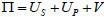(2)

When the pile buckling is solved by the Ritz method, the calculation must be done on the basis of the deformed system. When solving, an appropriate deflection function shall firstly be selected to obtain the internal energy and external work of the system; meanwhile, Ritz method requires that the corresponding displacement function satisfies the geometric boundary conditions.

The end-free bottom-fixing conditions are expressed as follows with reference to the deflection function of pile foundation under different boundary conditions [13M.A. Gabr, J.J. Wang, and M. Zhao, "Buckling of piles with general power distribution of lateral subgrade", J. Geotech. Geoenviron. Eng., vol. 123, pp. 123-130, 1997.
[http://dx.doi.org/10.1061/(ASCE)1090-0241(1997)123:2(123)]
], when x=0, y= 0 and y'=0; when x=h, y≠0 and y'≠ 0; the deflection function can be taken as: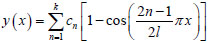(3)

Where: cn refers to undetermined coefficients.

According to the small elastic deformation theory, the elastic deformation energy, Us of the soil around pile is as follows: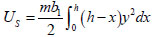(4)

The strain energy UP generated due to deflection of the corresponding pile is as follows:(5)

The potential energy V generated from the pile-top load is as follows:(6)

The total potential energy formula of the system obtained by substituting each potential energy equation into the total potential energy equation is as follows: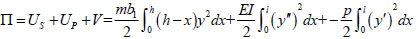(7)

Substitute the displacement function (3) into equation (7), after arrangement, the following equation is obtained: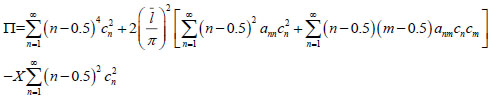(8)

Where: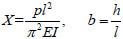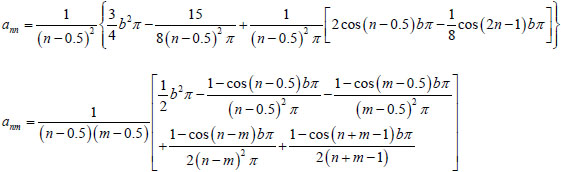Where: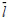refers to the dimensionless length of pile; b refers to the burying ratio of pile body.

According to the principle of stationary potential energy, take variation of equation (8) to obtain:(9)

Expand equation (9) to obtain:(10)

The above equation is a homogeneous linear equation of X. If only limited n items are taken from the approximate expression of pile displacement function y, then the equation can be written into matrix as follows:(11)

Where, bii=(i‒0.5)2+aiiA, bij=bji=aijA, A=2l-5π-5. The i and j values are substituted into ann and anm expressions, then the aii and aij expressions can be obtained.

To ensure that the equation set i.e. equation (11) has a non-zero solution, the coefficient determinant is required to be zero, namely: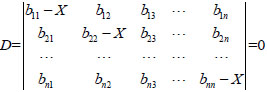(12)

Equation (12) is the characteristic equation of pile buckling stability. From the equation, the corresponding characteristic roots in number of n can be obtained with Jacobi method. Set the smallest positive root as xmin, the corresponding critical buckling load of pile Pcr can be obtained: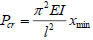(13)

Meanwhile, the pile buckling stability calculated length lP is found as follows: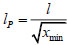(14)

During buckling analysis on piles in practical engineering, only displacement function half-wave numbers for one or several piles need to be taken to fit the flexural displacement function y of pile, then sufficient accurate solutions can be obtained.

As the static piles used in overall lifting is not particularly long, the half-wave number for pile deflection function is taken as n=1, then the displacement function can be taken as follows: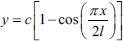(15)

The elastic deformation energy Us of the soil around pile can be obtained according to the small elastic deformation principle:(16)

The strain energy UP generated due to deflection of the corresponding pile is as follows:(17)

Also, the potential energy V generated from the pile-top load is obtained as follows:(18)

The total potential energy formula of the system obtained by substituting each potential energy equation into the total potential energy equation is as follows: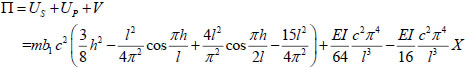(19)

According to the principle of stationary potential energy, take variation of the above equation to obtain:(20)

From equation (20), the following equation can be obtained:(21)

Then: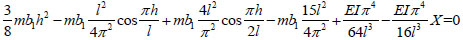(22)

The critical buckling load Pcr is as follows:(23)

Also the calculated length, lP for buckling stability of pile is as follows: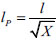(24)

From equation (22), we know that the calculated static pile length lP used for overall lifting are mainly influenced by factors e.g. the total length l of pile, free length lO of piles, buried depth h of pile, horizontal lateral resistance coefficient, m of soil and flexural rigidity EI of pile. As the geological conditions at the specific project location is definite, i.e. the horizontal lateral resistance coefficient m is a constant; and the size of the static pile used for this project is also unchanged, namely the flexural rigidity EI is unchanged, so only the law of change of the calculated length lP along with total length, l and free length lO of pile needs to be discussed in this paper.

layer of soil to the pile bottom(22~24), now take m=0, i.e. substitute into equations (22~24) without considering the influence of soil around pile and obtain lP = 2l. When the top is free and the bottom is fixed, the stability calculated length coefficient is 2 according to the classical Euler's formula. As can be seen, when the above calculation is not considered with the influence of soil around pile, the equation derived with Ritz method in this paper is reduced as the Euler's formula.

## 3. STABILITY ANALYSIS ON PILE FOUNDATION IN LAYERED ELASTIC FOUNDATION

In practical engineering, the soil around pile is in layered distribution rather than uniform distribution and the subgrade reaction coefficients distributed in each layer are different. Therefore, in order to analyze the problem veritably, this paper assumes that the soil is divided into n layers (Fig. 2).Fig. (2) Simplified analysis model for layered soil.

Terzaghi [14K. Terzaghi, "Evaluation of coefficients of subgrade reaction", Geotechnique, vol. 5, pp. 297-326, 1955.
[http://dx.doi.org/10.1680/geot.1955.5.4.297]
] expressed the horizontal subgrade reaction coefficient as: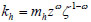(25)

Where: mh refers to variation coefficient of the subgrade reaction coefficient with depth; z refers to depth from surface; ω refers to empirical coefficient used to describe the foundation distribution pattern, non-negative; ζ refers to constant, for ease of standardization of dimension, it is recommended to be taken as 1 in literature [15P.W. Rowe, "The single pile subject to horizontal force", Geotechnique, vol. 6, pp. 70-85, 1956.
[http://dx.doi.org/10.1680/geot.1956.6.2.70]
];

From Equation (25), the reaction coefficient khi of ith layer of soil can be expressed as follows: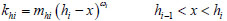(26)

Where: mhi is the coefficient for variation of the subgrade reaction coefficient of ith layer with depth; hi is the distance from ith layer of soil to the pile bottom, so hO=0; ωi=i power series for the ith layer of soil, ranging from 0 to 1.

The foundation horizontal reaction is expressed as equation [12The Third Railway Survey and Design Institute, Technical Manual of Railway Engineering Design(Foundation of Bridge), China Railway Publishing House: Beijing, 1991, pp. 1-48.]: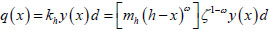(27)

Where: d is the pile diameter; x is the distance from the pile end; h is the buried depth of pile; and y is the horizontal deflection displacement of pile body.

Then, the distribution of the reaction of the soil around pile q with depth can be expressed as follows: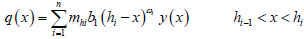(28)

Where: n is the total number of layers of foundation soil.

Similar to equations (4-7), the total potential energy of piles in layered elastic foundation is as follows:(29)

For convenience of calculation, assume that the certain reference subgrade reaction coefficient is khr, the subgrade reaction coefficient in ith layer of soil can be expressed as rikhr, then the above equation can be expressed as follows: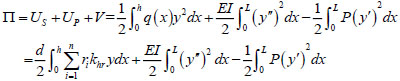(30)

Where, the deflection function y is as follows: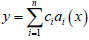(31)

From literature [12The Third Railway Survey and Design Institute, Technical Manual of Railway Engineering Design(Foundation of Bridge), China Railway Publishing House: Beijing, 1991, pp. 1-48.], the end free and bottom fixing conditions can be taken as follows: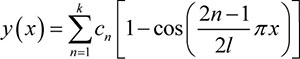According to the principle of stationary potential energy, take variation from the equation (30), namely: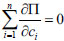(32)

Substitute equation (30) into equation (32) to obtain as follows: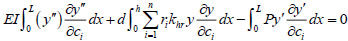(33)

Equation (33) can be expressed as follows:(34)

Where, [A] is the coefficient matrix and can be expressed as follows: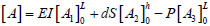(35)

Where: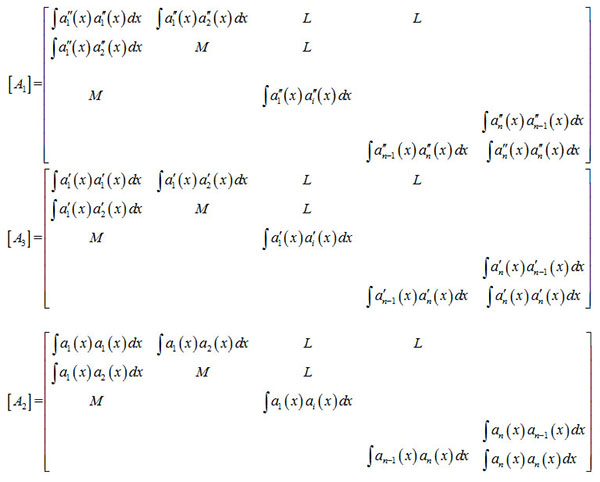By solving the coefficient matrix, the roots in number of n can be obtained. Set the smallest positive root as xmin and obtain the critical buckling load Pcr of corresponding pile as follows:(36)

Meanwhile, the calculated length LP for buckling stability of pile can be known as follows:(37)

## 4. EXAMPLES

In the lifting work of certain ancient masonry building, Φ194mm × 6mm small diameter opening static pile was used for lifting [9W. Lu, D. Zhao, and Y.L. Wang, "The key technology of integral lifting-up protection project of yuhuang pavilion-a review", Electronic Journal of Geotechnical Engineering, vol. 20, pp. 5009-5022, 2015.]. When pressing pile, the upper pile of the soil was in free length of 1.25m, the pile was in designed buried depth of 12m, and actual buried depth of 11~14m. Consider the calculated length of pile when the upper pile of the soil was in free length of 1.25m, and in buried depth of 12m.

### 4.1. Calculated Length of Pile in The Case of Uniform Foundation

Refer to literature [16(JGJ94-2008), “Technical Code for Building Pile Foundations, China Architecture and Building Press: Beijing, 2008, pp. 15-38.] for the value standard for subgrade reaction coefficient, and the physical and mechanical indicators of foundation soil are shown in Table 1 [17H.W. Feng, W. Lu, and P. Chen, "The key technology in rectification engineering by soil removal method of an inclined masonry pagoda", Electronic Journal of Geotechnical Engineering, vol. 21, pp. 1789-1802, 2016., 18H.W. Feng, "Estimation on pile driving resistance and soil plug evolution mechanism of the small-bore steel jacked pipe pile in silt foundation", Electronic Journal of Geotechnical Engineering, vol. 21, pp. 2949-2961, 2016.], so refer to Table 1 for the subgrade reaction coefficient values in each layer of soil:

Where, the value can be taken as m=15MN/m4; elastic modulus of steel E=2×105MPa, EI = 6.28 × 106N·m2. Substitute the above known conditions into Equation (11), and obtain X=7.87, the calculated length of the pile is: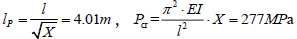### 4.2. Calculated Length of Pile in Case of Layered Foundation

Refer to Table 1 for the subgrade reaction coefficient values for each layer of soil; the steel pipe pile parameters are the same as the former; substitute the above known conditions into Equation (34) and obtain xmin = 6.11, then the calculated length of pile is as follows: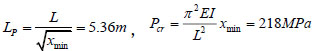### 4.3. Comparison of Results

Compared with the calculation results of the uniform foundation model, the calculated length of layered foundation model is increased by 25%, and the buckling load is reduced by 27%. It shows that the pile body is more prone to buckling under the layered soil foundation model.

## 5. COMPARISON OF ALGORITHMS

Engineering condition same as section 4, the total length of pile is 13.25m, designed buried depth of 12m and the upper pile of the soil was in free length of 1.25m. With the increase of buried depth of pile, the change trend of the buckling load as shown in Fig. (3).Fig. (3) Relationship between Buried Depth and Buckling Load.

As shown in Fig. (3), buckling load indicates the relationship between field measured buckling load and pile buried depth, ‘m’ method indicates the relationship between the buckling load calculated by the ‘m’ method and pile buried depth.

The measured results show that, with the increase of the buried depth, the buckling load increased significantly, but the buckling load increases slowly when the buckling depth exceeds 6 m, which is stable at 230MPa or so. Compared with the measured results, the calculated result of “m” method is conservative, and it has a great space for optimization. The curve shape which calculated by uniform foundation modal is similar to that of the measured, but the buckling value is 10% larger than measured. The calculated result of layered soil model is in good agreement with the measured data, but the calculation value of buckling load is lower than measured data in the initial stage and obviously larger when buried depth exceeds 6m. Therefore, the layered foundation model proposed in this paper can better simulate the real situation and obtaining accurate buckling loads.

## CONCLUSION

This paper made an analysis on the stability of piles in uniform foundation and layered foundation based on the energy method and described the characteristics of the influences from the soil surrounding pile. The constraints of piles were simplified as bottom fixing upper free. Taking into account the influence of reaction of soil around pile, the calculated length of steel pipe pile was analyzed, and the equations on calculated length of piles in uniform foundation and layered foundation are obtained, and the application of the equations was explained in combination with examples. These equations have advantages of simple form and definitive meanings for parameter, thus easy to use, and can provide certain reference value in determining calculated length of pile on similar foundation works.

### CONFLICT OF INTEREST

The authors confirm that this article content has no conflict of interest.

Declared none.

## REFERENCES

  Q. Jia, H.Q. Ying, and X. Zhang, "Construction of basement in existing building by static bolt-pile", Rock and Soil Mechanics, vol. 30, pp. 2053-2057, 2009.  B. Juergen, and B. Dietrich, "Buckling lengths of freestanding piles", Bautechnik, vol. 66, pp. 375-382, 1989.  R.L. Hu, Analysis and Design of Bridge Pile Foundation, China Railway Publishing House: Beijing, 1987, pp. 266-275.  L. Chi-wai, "Parametric studies on buckling of piles in cohesionless soils by numerical methods", HKIE Transactions Hong Kong Institution of Engineers, vol. 20, pp. 12-33, 2013.  X.J. Zou, M.H. Zhao, and G.D. Liu, "Buckling analysis of cast-in-situ rock-socketed piles with highrise pile cap under complicated subgrade reaction modulus", Rock and Soil Mechanics, vol. 29, pp. 963-967, 2008.  H.F. Shan, T.D. Xia, and J.H. Hu, "Buckling stability analysis of pile foundation for excavation beneath the basement of existing building", Rock and Soil Mechanics, vol. 36, pp. 507-512, 2015.  Q.Z. Jia, A.P. Zheng, and X. Zhang, "Test study and numerical analysis of stability of high-level cap steel tubular pile under compression loads", Rock and Soil Mechanics, vol. 32, pp. 1736-1740, 2011.  W.H. Yang, and L. Song, "Aaxial buckling analysis for top-free and bottom-fixed pile", Engineering Mechanics, vol. 17, pp. 36-66, 2000.  W. Lu, D. Zhao, and Y.L. Wang, "The key technology of integral lifting-up protection project of yuhuang pavilion-a review", Electronic Journal of Geotechnical Engineering, vol. 20, pp. 5009-5022, 2015.  M.H. Zhao, and J.B. Wang, "Buckling analysis of bouyant piles", Journal of Hunan University, vol. 22, pp. 109-114, 1995.  M.H. Zhao, and J.B. Wang, "Buckling analysis of pile considering frictional resistance", Chinese Journal of Geotechnical Engineering, vol. 18, pp. 87-90, 1996.  The Third Railway Survey and Design Institute, Technical Manual of Railway Engineering Design(Foundation of Bridge), China Railway Publishing House: Beijing, 1991, pp. 1-48.  M.A. Gabr, J.J. Wang, and M. Zhao, "Buckling of piles with general power distribution of lateral subgrade", J. Geotech. Geoenviron. Eng., vol. 123, pp. 123-130, 1997.[http://dx.doi.org/10.1061/(ASCE)1090-0241(1997)123:2(123)]  K. Terzaghi, "Evaluation of coefficients of subgrade reaction", Geotechnique, vol. 5, pp. 297-326, 1955.[http://dx.doi.org/10.1680/geot.1955.5.4.297]  P.W. Rowe, "The single pile subject to horizontal force", Geotechnique, vol. 6, pp. 70-85, 1956.[http://dx.doi.org/10.1680/geot.1956.6.2.70]  (JGJ94-2008), “Technical Code for Building Pile Foundations, China Architecture and Building Press: Beijing, 2008, pp. 15-38.  H.W. Feng, W. Lu, and P. Chen, "The key technology in rectification engineering by soil removal method of an inclined masonry pagoda", Electronic Journal of Geotechnical Engineering, vol. 21, pp. 1789-1802, 2016.  H.W. Feng, "Estimation on pile driving resistance and soil plug evolution mechanism of the small-bore steel jacked pipe pile in silt foundation", Electronic Journal of Geotechnical Engineering, vol. 21, pp. 2949-2961, 2016.

### Endorsements

"Open access will revolutionize 21st century knowledge work and accelerate the diffusion of ideas and evidence that support just in time learning and the evolution of thinking in a number of disciplines."

Daniel Pesut
(Indiana University School of Nursing, USA)

"It is important that students and researchers from all over the world can have easy access to relevant, high-standard and timely scientific information. This is exactly what Open Access Journals provide and this is the reason why I support this endeavor."

Jacques Descotes
(Centre Antipoison-Centre de Pharmacovigilance, France)

"Publishing research articles is the key for future scientific progress. Open Access publishing is therefore of utmost importance for wider dissemination of information, and will help serving the best interest of the scientific community."

Patrice Talaga
(UCB S.A., Belgium)

"Open access journals are a novel concept in the medical literature. They offer accessible information to a wide variety of individuals, including physicians, medical students, clinical investigators, and the general public. They are an outstanding source of medical and scientific information."

Jeffrey M. Weinberg
(St. Luke's-Roosevelt Hospital Center, USA)

"Open access journals are extremely useful for graduate students, investigators and all other interested persons to read important scientific articles and subscribe scientific journals. Indeed, the research articles span a wide range of area and of high quality. This is specially a must for researchers belonging to institutions with limited library facility and funding to subscribe scientific journals."

Debomoy K. Lahiri
(Indiana University School of Medicine, USA)

"Open access journals represent a major break-through in publishing. They provide easy access to the latest research on a wide variety of issues. Relevant and timely articles are made available in a fraction of the time taken by more conventional publishers. Articles are of uniformly high quality and written by the world's leading authorities."

Robert Looney
(Naval Postgraduate School, USA)

"Open access journals have transformed the way scientific data is published and disseminated: particularly, whilst ensuring a high quality standard and transparency in the editorial process, they have increased the access to the scientific literature by those researchers that have limited library support or that are working on small budgets."

Richard Reithinger
(Westat, USA)

"Not only do open access journals greatly improve the access to high quality information for scientists in the developing world, it also provides extra exposure for our papers."

J. Ferwerda
(University of Oxford, UK)

"Open Access 'Chemistry' Journals allow the dissemination of knowledge at your finger tips without paying for the scientific content."

Sean L. Kitson
(Almac Sciences, Northern Ireland)

"In principle, all scientific journals should have open access, as should be science itself. Open access journals are very helpful for students, researchers and the general public including people from institutions which do not have library or cannot afford to subscribe scientific journals. The articles are high standard and cover a wide area."

Hubert Wolterbeek
(Delft University of Technology, The Netherlands)

"The widest possible diffusion of information is critical for the advancement of science. In this perspective, open access journals are instrumental in fostering researches and achievements."

Alessandro Laviano
(Sapienza - University of Rome, Italy)

"Open access journals are very useful for all scientists as they can have quick information in the different fields of science."

Philippe Hernigou
(Paris University, France)

"There are many scientists who can not afford the rather expensive subscriptions to scientific journals. Open access journals offer a good alternative for free access to good quality scientific information."

Fidel Toldrá
(Instituto de Agroquimica y Tecnologia de Alimentos, Spain)

"Open access journals have become a fundamental tool for students, researchers, patients and the general public. Many people from institutions which do not have library or cannot afford to subscribe scientific journals benefit of them on a daily basis. The articles are among the best and cover most scientific areas."

M. Bendandi
(University Clinic of Navarre, Spain)

"These journals provide researchers with a platform for rapid, open access scientific communication. The articles are of high quality and broad scope."

Peter Chiba
(University of Vienna, Austria)

"Open access journals are probably one of the most important contributions to promote and diffuse science worldwide."

Jaime Sampaio
(University of Trás-os-Montes e Alto Douro, Portugal)

"Open access journals make up a new and rather revolutionary way to scientific publication. This option opens several quite interesting possibilities to disseminate openly and freely new knowledge and even to facilitate interpersonal communication among scientists."

Eduardo A. Castro
(INIFTA, Argentina)

"Open access journals are freely available online throughout the world, for you to read, download, copy, distribute, and use. The articles published in the open access journals are high quality and cover a wide range of fields."

Kenji Hashimoto
(Chiba University, Japan)

"Open Access journals offer an innovative and efficient way of publication for academics and professionals in a wide range of disciplines. The papers published are of high quality after rigorous peer review and they are Indexed in: major international databases. I read Open Access journals to keep abreast of the recent development in my field of study."

Daniel Shek
(Chinese University of Hong Kong, Hong Kong)

"It is a modern trend for publishers to establish open access journals. Researchers, faculty members, and students will be greatly benefited by the new journals of Bentham Science Publishers Ltd. in this category."

Jih Ru Hwu
(National Central University, Taiwan)

Webmaster Contact: info@benthamopen.net
Copyright © 2019 Bentham Open